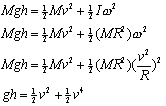Teaching Physics with the Physics Suite Edward F. Redish

Finding a Mistake using Dimensional Analysis

As part of an examination a few years ago, a student went through the algebraic manipulations on an exam shown in the figure at the right below. At this point you don't know what the symbols mean, but given the information at the left about the dimensions associated with each symbol, decide

1. Is it possible that the final equation is correct?
2. If the final equation is not correct, does that mean that the starting equation necessarily wrong?
3. If the final equation is not correct and the starting equation is not wrong, can you find the error using dimensional analysis?
 [M] = M [g]=L/T2 [h]=L [w]=1/T [v]=L/T [R]=L [I]=ML2Not finding what you wanted? Check the Site Map for more information.